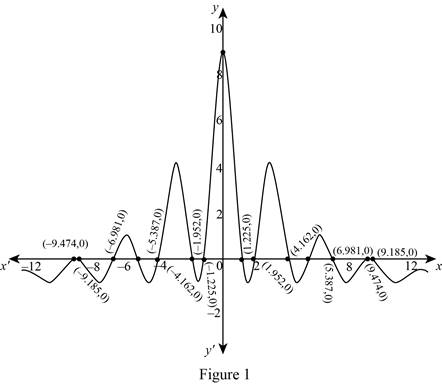# The number of critical numbers.### Single Variable Calculus: Concepts...

4th Edition
James Stewart
Publisher: Cengage Learning
ISBN: 9781337687805### Single Variable Calculus: Concepts...

4th Edition
James Stewart
Publisher: Cengage Learning
ISBN: 9781337687805

#### Solutions

Chapter 4.2, Problem 40E
To determine

## To find: The number of critical numbers.

Expert Solution

The function f has 14 critical numbers.

### Explanation of Solution

Given:

A formula for the derivative of a function f(x)=100cos2x10+x21 .

Calculation:

Since the function is defined on , it is enough to identify the number of the zeros.

Draw the graph of f'(x) and identify the number of zeros.

Use the graphic calculator and draw the graph of f'(x) as shown below in Figure 1.From Figure 1, it is observed that the graph has 10 x-intercepts. Hence, there are 14 zeros.

Thus, the function f(x) has 14 critical numbers.

### Have a homework question?

Subscribe to bartleby learn! Ask subject matter experts 30 homework questions each month. Plus, you’ll have access to millions of step-by-step textbook answers!